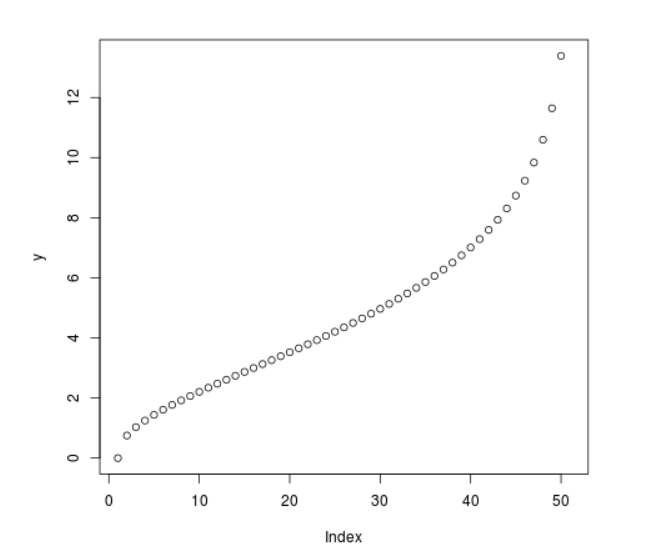Related Articles
Compute value of Quantile Chi Square Density in R Programming – qchisq() Function
• Last Updated : 25 Jun, 2020

`qchisq()` function in R Language is used to compute value of chi square quantile function. It creates a quantile function plot for chi square distribution.

Syntax: qchisq(vec, df)

Parameters:
vec: Vector of x-values
df: Degree of Freedom

Example 1:

 `# R program to compute  ` `# Quantile Chi Square density ` ` `  `# Create a vector of x-values ` `x <``-` `seq(``0``, ``1``, by ``=` `0.1``) ` ` `  `# Calling qchisq() Function ` `y <``-` `qchisq(x, df ``=` `5``) ` `y `

Output:

```  0.000000 1.610308 2.342534 2.999908 3.655500 4.351460 5.131867 6.064430
 7.289276 9.236357      Inf
```

Example 2:

 `# R program to compute  ` `# Quantile Chi Square density ` ` `  `# Create a vector of x-values ` `x <``-` `seq(``0``, ``1``, by ``=` `0.02``) ` ` `  `# Calling qchisq() Function ` `y <``-` `qchisq(x, df ``=` `5``) ` ` `  `# Plot a graph ` `plot(y) `

Output:My Personal Notes arrow_drop_up
Recommended Articles
Page :